# Python 数据挖掘与机器学习实战（二）：Python 语言简介## 2.1　搭建 Python 开发环境

Python 可应用于多平台，包括 Linux 和 Mac OSX。本节主要介绍如何在 Windows 平台搭建 Python 开发环境，以及运行和保存相应的 Python 程序。

### 2.1.1　安装 Anaconda

Anaconda 是 Python 的一个开源发行版本，主要面向科学计算，内含有诸多机器学习算法框架、图像处理模块及经典算法集成模块。Anaconda 的主要优点是预装了很多第三方库，而且增加了 conda install 命令，除了使得安装新的 package 非常方便外，还自带了 Spyder IDE 和 Jupyter Notebook 等编译环境。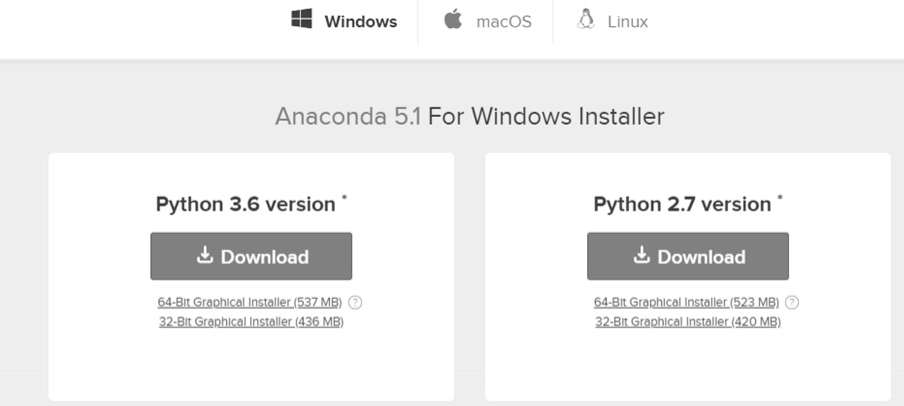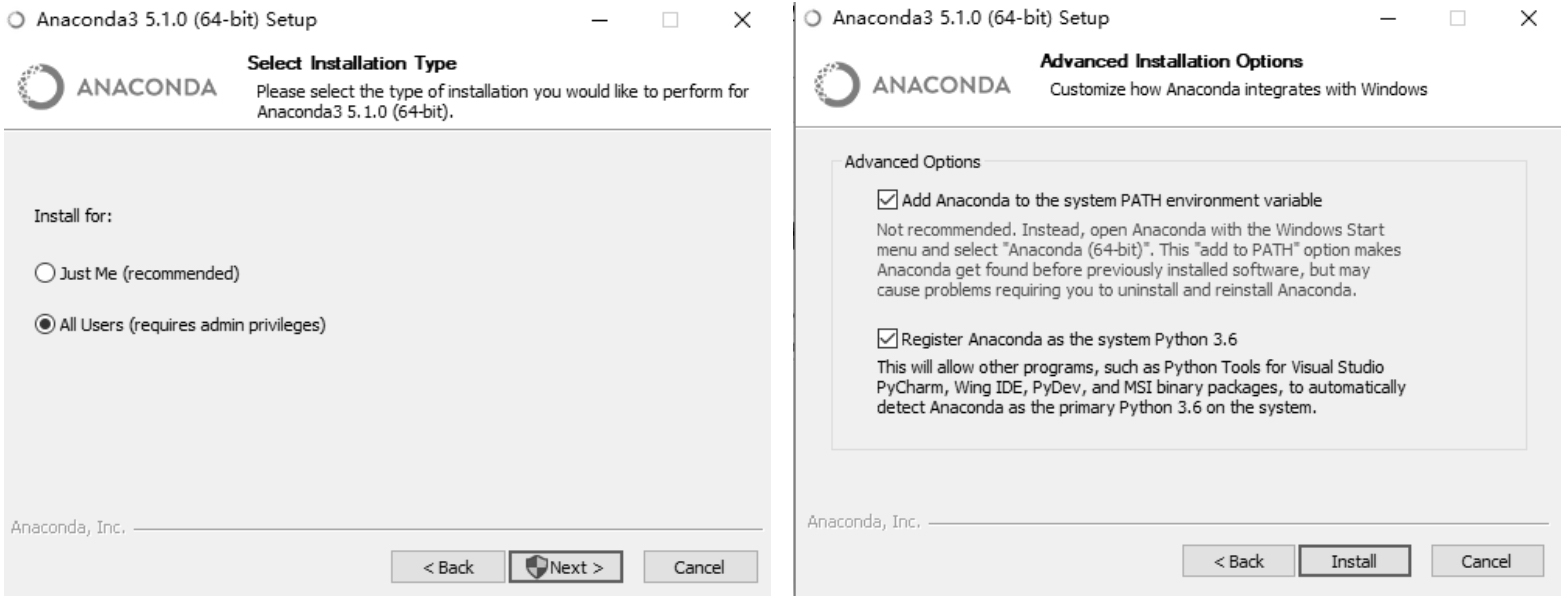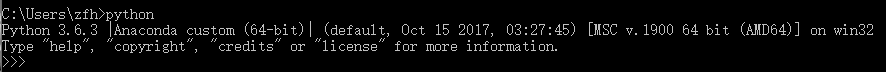### 2.1.2　安装 Spyder

Spyder 是 Python 的作者为 Python 开发的一个简单的集成开发环境。和其他的 Python 开发环境相比，Spyder 的最大优点就是模仿 MATLAB 的“工作空间”的功能，可以很方便地观察和修改数组的值。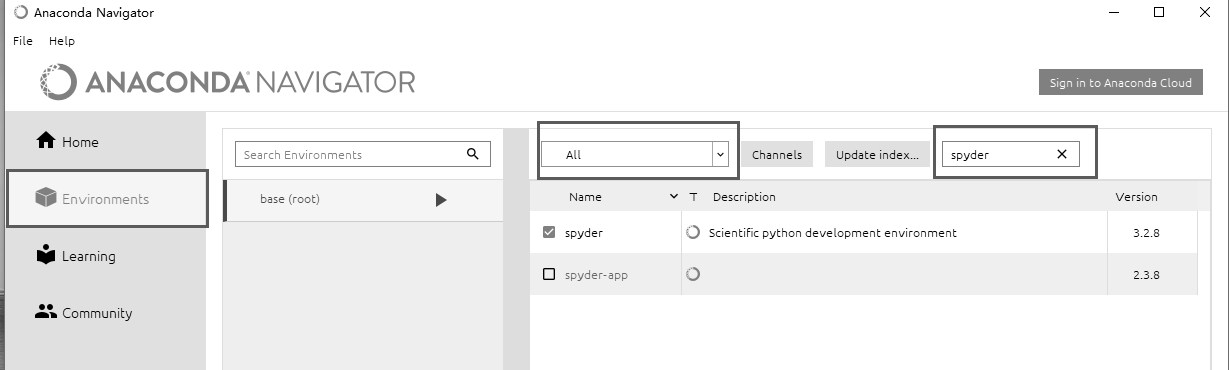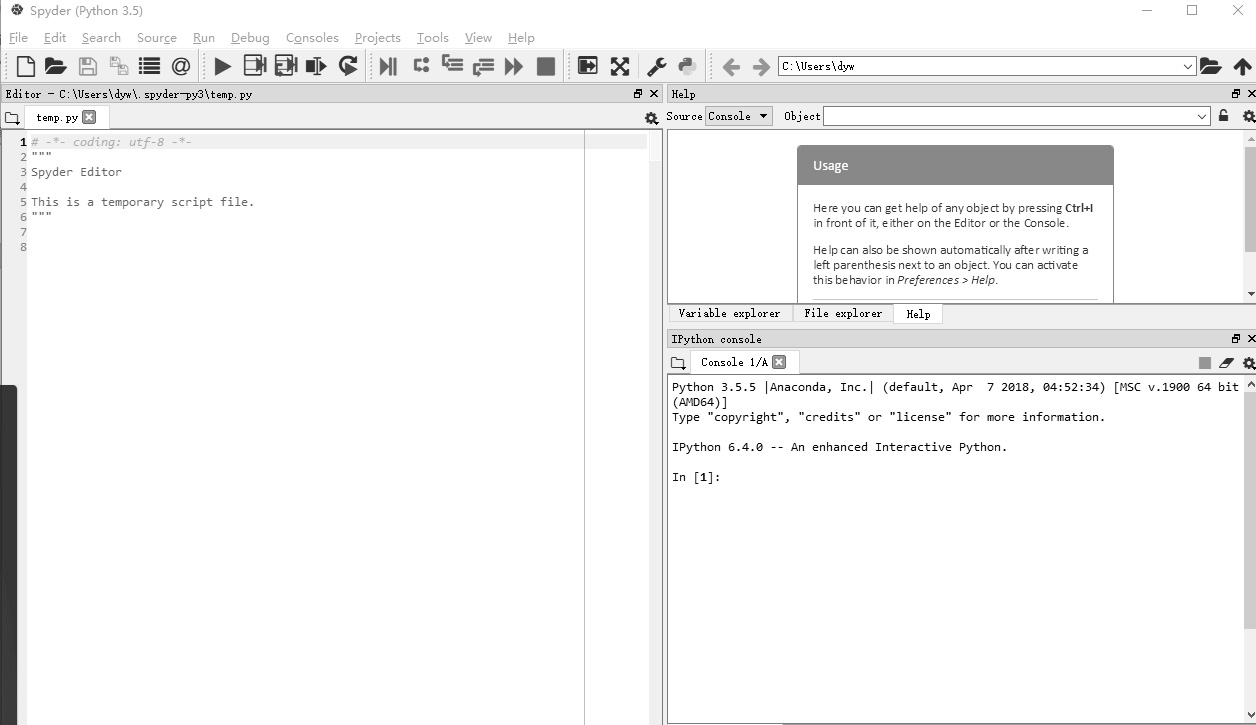### 2.1.3　运行和保存 Python 程序

print("Hello World")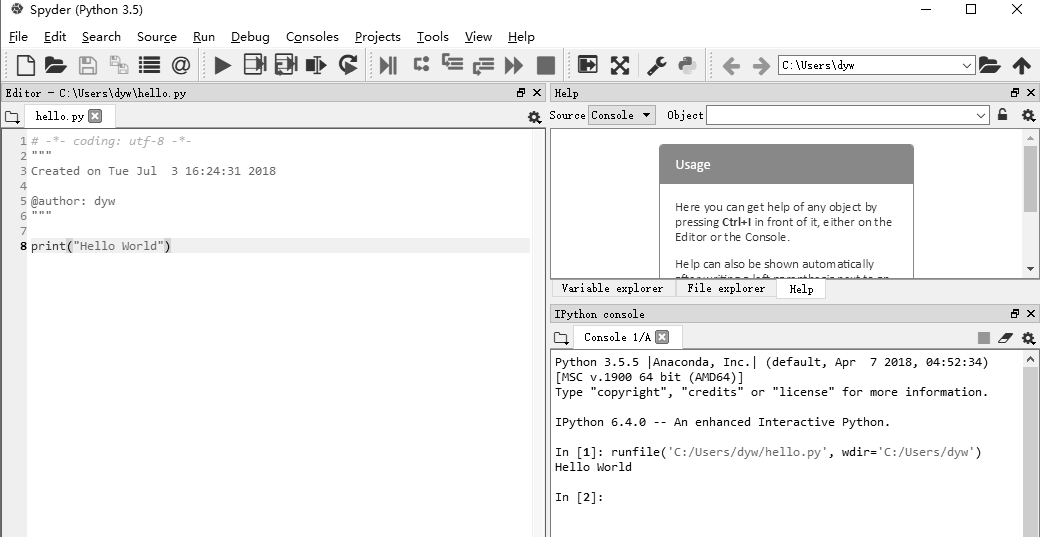## 2.2　Python 计算与变量

Python 开发环境已经搭建完成，也知道如何运行和保存程序了，现在就可以使用它来编写自己的程序了。本节首先从一些基本的数学运算开始讲解，然后再使用变量进行稍复杂一些的计算。变量是程序中用来保存东西（如数值和矩阵等内容）的一种方式，它们能使程序更加简单明了。

### 2.2.1　用 Python 做简单的计算

（1）单击“开始”按钮，输入 cmd，进入命令行窗口。

（2）再输入 python，然后按 Enter 键，即进入 Python 编辑环境。

（3）显示当前的 Python 版本。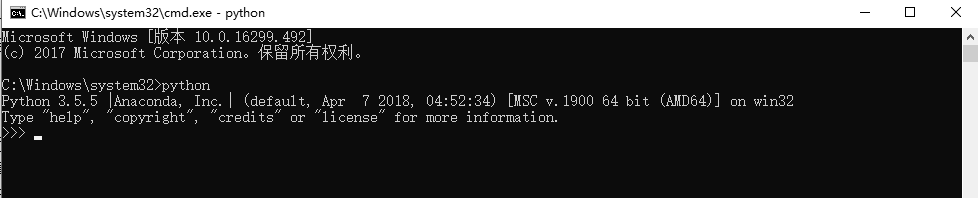>>> 9*8.4676.14

### 2.2.2　Python 的运算符

Python 中用斜杠“/”来表示除法，因为这与写分数的方式相似。例如，a=20，b=10，在 Python 程序中计算 a 除以 b，只要输入 20/10，输出结果为 2。要记住“斜杠”是顶部靠右的那个（顶部靠左的是反斜杠“\”)。“%”表示取模，即返回除法的余数，如 a%b 的输出结果是 0。“”表示幂，即返回 x 的 y 次幂，ab 是 10 的 20 次方，输出是 100000000000000000000。

+
-
*
/
% 取模
// 向下取整
**

### 2.2.3　Python 的变量

Python 中的变量赋值不需要类型声明。在内存中创建每个变量时包括了变量的标识、名称和数据这些信息。每个变量在使用前都必须赋值，变量赋值以后该变量才会被创建。等号“=”用来给变量赋值，其运算符左边是一个变量名，右边是存储在变量中的值。例如：

counter = 1000                                                     #赋值整型变量miles = 1000.0                                                     #浮点型str = "Python"                                                     #字符串print (counter)print (miles)print (str)

10001000.0Python

Python 允许同时为多个变量赋值。例如：

a = b = c = 1000

a, b, c = 1, 2, "abc"

## 2.3　Python 的字符串

str1 = 'Hello World!'str2 = "Python"print(str1)print(str2)

Hello World!Python

Python 不支持单字符类型，单字符在 Python 中也是作为一个字符串使用的。在 Python 中访问子字符串时，可以使用方括号“[]”来截取字符串，例如：

str1 = 'Hello World!'str2 = "Python"print ("str1: ", str1)print ("str2[1:5]: ", str2[1:5])

str1: Hstr2[1:5]: ytho

str1 = 'Hello 'str2 = 'world!'str1 = str1+str2print(str1)

Hello world!

+ 字符串连接
* 重复输出字符串
[] 通过索引获取字符串中的字符
[ : ] 截取字符串中的一部分
in 如果字符串中包含给定的字符则返回 True
not in 如果字符串中不包含给定的字符则返回 True
% 格式字符串

a = "Hello"b = "Python"print ("a + b 输出结果：", a + b)print ("a * 2 输出结果：", a * 2)print ("a 输出结果：", a)print ("a[1:4] 输出结果：", a[1:4])if( "H" in a) :    print ("H 在变量 a 中 ")else :    print ("H 不在变量 a 中 ")if( "N" not in a) :    print ("N 不在变量 a 中 ")else :print ("N 在变量 a 中 ")

a + b 输出结果： HelloPythona * 2 输出结果： HelloHelloa 输出结果： ea[1:4] 输出结果： ellH 在变量 a 中N 不在变量 a 中

Python 支持格式化字符串的输出。尽管这样可能会用到非常复杂的表达式，但最基本的用法是将一个值插入到一个有字符串格式符“%s”的字符串中。在 Python 中，字符串格式化使用与 C 语言中 printf，函数的语法一样。例如：

print ("My name is %s and age is %d!" % ('xiaoming', 20))

My name is xiaoming and age is 20!

## 2.4　Python 的列表

list1 = ['a', 'b', 10, 20]list2 = [1, 2, 3, 4]list3 = ["a", "b", "c"]

list1 = ['a', 'b', 10, 20]list2 = [1, 2, 3, 4]print ("list1: ", list1)print ("list2[1:4]: ", list2[1:4])

list1:  alist2[1:4]:  [2, 3, 4]

list = []                                        #空列表list.append('Hello')                             #使用 append() 添加元素list.append('World!')print (list)

['Hello', 'World!']

list1 = ['a', 'b', 10, 20]print (list1)del list1print (" 删除后的输出为 : ")print (list1)

['a', 'b', 10, 20]删除后的输出为 :['a', 'b', 20]

Python 列表操作符和字符串操作符有些是相似的，如“+”号用于组合列表，“*”号用于重复列表。如表 2-3 所示为常见的列表操作符。

len 列表长度
+ 组合
* 重复
in 元素是否存在于列表中
for 迭代

list1 = [1,2,3]list2 = [4,5,6]print(len(list1))print(list1+list2)print(list1*3)print(3 in list1)for x in list1:    print (x)

3[1, 2, 3, 4, 5, 6][1, 2, 3, 1, 2, 3, 1, 2, 3]True123

## 2.5　Python 的元组

Python 的元组与列表类似，不同之处在于元组的元素不能修改；元组使用小括号，列表使用方括号。元组的创建很简单，只需要在括号中添加元素，并使用逗号隔开即可。例如：

tup1 = ('a', 'b', 10, 20)tup2 = (1, 2, 3, 4, 5 )tup3 =("a", "b", "c", "d")tup1 = ()                                                       #创建空元组

tup1 = (50,)

tup1 = ('a', 'b', 10, 20)tup2 = (1, 2, 3, 4, 5 )print ("tup1: ", tup1)print ("tup2[1:4]: ", tup2[1:4])

tup1:  atup2[1:4]:  (2, 3, 4)

tup1 = (12, 34.56)tup2 = ('abc', 'xyz')# 以下修改元组元素操作是非法的# tup1 = 100# 创建一个新的元组tup3 = tup1 + tup2print (tup3)

(12, 34.56, 'abc', 'xyz')

tup1 = ('a', 'b', 10, 20)print (tup)del tupprint (" 删除后的结果: ")print (tup)

 ('a', 'b', 10, 20)删除后的结果:Traceback (most recent call last):  File "F:/program/2.5.py", line 26, in <module>    print (tup)NameError: name 'tup' is not defined

len 计算元素个数
+ 连接
* 复制
in 元素是否存在
for 迭代

tup1 = (1,2,3)tup2 = (4,5,6)print(len(tup1))print(tup1+tup2)print(tup1*3)print(3 in tup1)　for x in tup1:    print (x)

3(1, 2, 3, 4, 5, 6)(1, 2, 3, 1, 2, 3, 1, 2, 3)True123

## 2.6　Python 的字典

dict = {key1 : value1, key2 : value2 }

dict = {'a': 1, 'b': 2, 'b': '3'}print(dict['b'])print(dict)

3{'a': 1, 'b': '3'}

dict = {'Alice': '20', 'Beth': '21', 'Cecil': '22'}

dict1 = { 'abc':123}dict2 = { 'abc': 123, 98: 37 }

dict = {'Name': 'xioaming', 'Age': 20, 'Class': 'First'}print ("dict['Name']: ", dict['Name'])print ("dict['Age']: ", dict['Age'])

dict['Name']:  xioamingdict['Age']:  20

dict = {'Name': 'xioaming', 'Age': 20, 'Class': 'First'}print ("dict[xiaowang']: ", dict['xiaowang'])

Traceback (most recent call last):  File "F:/program/2.6.py", line 19, in <module>    print ("dict[xiaowang']: ", dict['xiaowang'])KeyError: 'xiaowang'

dict = {'Name': 'xioaming', 'Age': 20, 'Class': 'First'}dict['Age'] = 22                        #修改年龄dict['School'] = "NUIST"                 #添加新的键 / 值对print ("dict['Age']: ", dict['Age'])print ("dict['School']: ", dict['School'])

dict['Age']:  22dict['School']:  NUIST

dict = {'Name': 'xioaming', 'Age': 20, 'Class': 'First'}del dict['Name']                     #删除键是'Name'的条目dict.clear()                         #清空词典所有条目del dict                            #删除词典print ("dict['Age']: ", dict['Age'])print ("dict['School']: ", dict['School'])

Traceback (most recent call last):  File "F:/program/DCGAN-tensorflow-master/2.6.py", line 34, in <module>    print ("dict['Age']: ", dict['Age'])TypeError: 'type' object is not subscriptable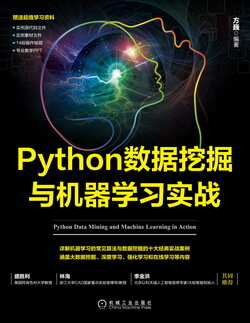Python 数据挖掘与机器学习实战（一）：Python 语言优势及开发工具

Python 数据挖掘与机器学习实战（二）：Python 语言简介

Python 数据挖掘与机器学习实战（三）：网络爬虫原理与设计实现

Python 数据挖掘与机器学习实战（四）：用 Python 实现多元线性回归

Python 数据挖掘与机器学习实战（五）：基于线性回归的股票预测